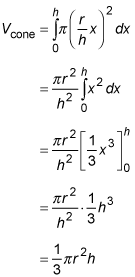##### Calculus Workbook For Dummies with Online PracticeIn addition to finding the volume of unusual shapes, integration can help you to derive volume formulas. For example, you can use the disk/washer method of integration to derive the formula for the volume of a cone.

Integration works by cutting something up into an infinite number of infinitesimal pieces and then adding the pieces up to compute the total. The disk/washer method cuts up a given shape into thin, flat disks or washers; this makes it useful for shapes with circular cross-sections, like, well, cones.

The following practice question asks you to apply the disk method for just this purpose.

## Practice question

1. Use the disk method to derive the formula for the volume of a cone. Hint: What's your function? See the following figure. Your formula should be in terms of r and h.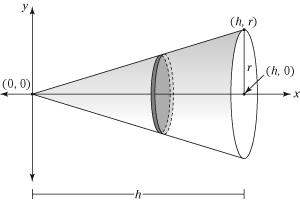1. The formula is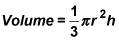How do you get it? First, find the function that revolves about the x-axis to generate the cone.

The function is the line that goes through (0, 0) and (h, r). Its slope is thus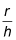and its equation is therefore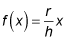Now express the volume of a representative disk. The radius of your representative disk is f (x) and its thickness is dx. Thus, its volume is given by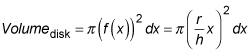Finally, add up the disks from x = 0 to x = h by integrating. (Don't forget that r and h are constants that behave like numbers.)